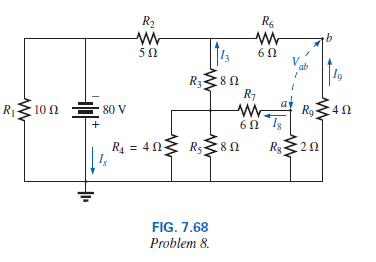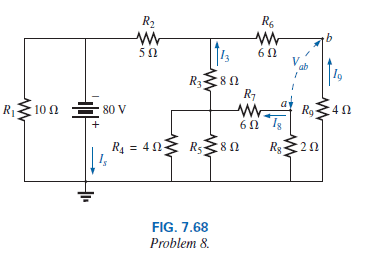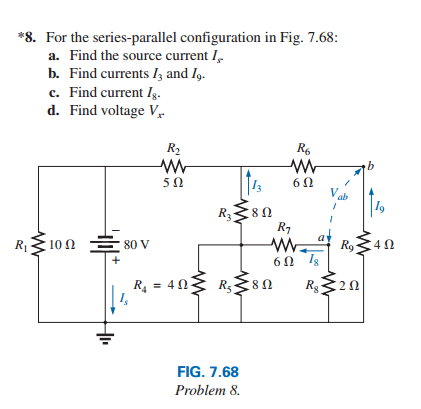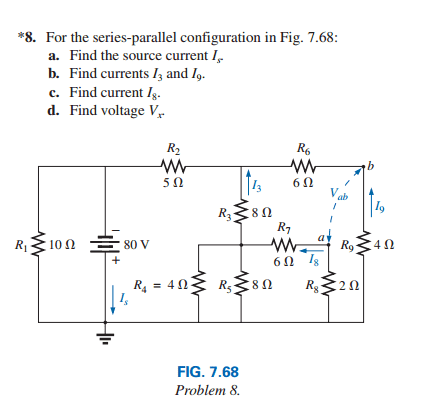# Which Law should i use to analyze this circuit ?

• Engineering
Which Law should i use to analyze this circuit ??

Hello Guys !!

Sorry i have many questions because iam not a professional in this ..

My Question is :

in this photo i found that the total Resistance is : 5 ohm
and
the total current is 16 A
and
I3 = I9 = 4 A
and I8= 1 A

I can't understand how to count I8 and Which Law should be used to get it ??Thanks Guys !!Last edited:

berkeman
Mentor
Hello Guys !!

Sorry i have many questions because iam not a professional in this ..

My Question is :

in this photo i found that the total Resistance is : 5 ohm
and
the total current is 16 A
and
I3 = I9 = 4 A
and I8= 1 A

I can't understand how to count I8 and Which Law should be used to get it ??Thanks Guys !!How did you come to believe that the total resistance is 5 Ohms? Resistance between where and where?

And how did you calculate those currents? Please show us your work in detail.

I would use KCL to analyze this circuit. Can you write out the KCL equations and show us how you solve them simultaneously?

okay ..

R′= R4 || R5 ||(R7+ R8) = 4 Ω ||8 Ω ||(6 Ω+ 2 Ω) = 4 Ω ||8 Ω ||8 Ω= 4 Ω ||4 Ω= 2 Ω
R′ ′ = (R3+ R′) ||(R6+ R9) = (8 Ω+ 2 Ω) ||(6 Ω+ 4 Ω) = 10 Ω ||10 Ω= 5 Ω
RT= R1 ||(R2+R′ ′ ) = 10 Ω ||(5 Ω+ 5 Ω) = 10 Ω ||10 Ω= 5 Ω

I=E/RT = 80/5=16A
I across R2=I/2 =8A
I3 = I9 = 4A

Then I8 is written to be 1 A
but i don't know how to get it

berkeman
Mentor
okay ..

R′= R4 || R5 ||(R7+ R8) = 4 Ω ||8 Ω ||(6 Ω+ 2 Ω) = 4 Ω ||8 Ω ||8 Ω= 4 Ω ||4 Ω= 2 Ω
R′ ′ = (R3+ R′) ||(R6+ R9) = (8 Ω+ 2 Ω) ||(6 Ω+ 4 Ω) = 10 Ω ||10 Ω= 5 Ω
RT= R1 ||(R2+R′ ′ ) = 10 Ω ||(5 Ω+ 5 Ω) = 10 Ω ||10 Ω= 5 Ω

I=E/RT = 80/5=16A
I across R2=I/2 =8A
I3 = I9 = 4A

Then I8 is written to be 1 A
but i don't know how to get it

What is the exact problem statement? What equivalent resistance are you asked to find?

okay .. this is the exact question and the exact statments which are requiredi want to know how to get I8 because i don't know which law should i use to find ??

berkeman
Mentor
okay .. this is the exact question and the exact statments which are requiredi want to know how to get I8 because i don't know which law should i use to find ??

In general, you use the KCL equations to solve a circuit like this. You cannot always rely on series/parallel combinations to solve this type of question.

Are you familiar with how to write KCL equations? Have you learned them yet?

We Didn't take applications on KCL yet but i tried to apply it on simple circuits and i found it easy

but i don't know how to apply it on this circuit so please tell meberkeman
Mentor
We Didn't take applications on KCL yet but i tried to apply it on simple circuits and i found it easy

but i don't know how to apply it on this circuit so please tell mehttp://en.wikipedia.org/wiki/Kirchhoff's_current_law#Kirchhoff.27s_current_law_.28KCL.29

Don't worry about the integral stuff near the beginning of the article. Focus on the simpler algebraic equations. You basically go to each node in the schematic, and you write an equation for that node that lists all of the currents going out of the node. You then solve all of those equations simultaneously to find all of the currents, and then use the currents to find any voltages that you need.

Have a look at the article and see if that helps.•1 person
okay iam trying to apply KCL by myself so please tell me

am i right or not ??

I3 = I4 + I5 +I7 + I8
so
4 A = 0.5 I8 + 0.25 I8 + (1/3) I8 +I8
so
I8 will be 3.7 A

i feel that i'm very stupid :shy:

i don't know the right way to apply KCL here

berkeman
Mentor
okay iam trying to apply KCL by myself so please tell me

am i right or not ??

I3 = I4 + I5 +I7 + I8
so
4 A = 0.5 I8 + 0.25 I8 + (1/3) I8 +I8
so
I8 will be 3.7 A

i feel that i'm very stupid :shy:

i don't know the right way to apply KCL here

To start writing the KCL equations, you must give each node a number. Call the bottom ground rail node 0. Call the top (negative terminal) of the voltage source node 1. Call the node to the right of R2 node 2. Call the node beloow R3 node 3. The two resistors on the right shouldn't be combined if they are asking about Vab, so call the node to the right of R6 node 4.

Then you write the resistor currents as the difference in the voltage across the resistor divided by the resistance. So you would write the KCL equation for node 1 like this:

-80V/R1 + I5 + (-80V - V2)/R2 = 0

Write the other KCL equations for nodes 2-4, and then solve the 4 equations for the unknown voltages. Once you have the voltages at each node in the circuit, you can calculate all the currents.

epenguin
Homework Helper
Gold Member
I don't know how you can get the answers you got and then not this. I think I have got it without writing anything down because they have used nice numbers that simplify it. You just need to use the fact that current divides itself between parallel branches in proportion to the conductance ( reciprocal of resistance) of the branch.

If I am not mistaken the current divides itself equally between the left and the right circuits. In the right circuit it again divides itself equally between the two branches after R2 (easier to think about the clockwise flow). So 4A flows through the R3 branch. That again divides itself half and half between the left resistor and the right pair, which again are equal, so 1A flows through the R7,8 branch.

Well check that, but use the principle I mentioned.

In general currents won't divide thenselves half-and-half as they did here because of the particular values of the conductances, but you can use whatever ratio the conductances of the parallel branches have.

Last edited:
•1 person